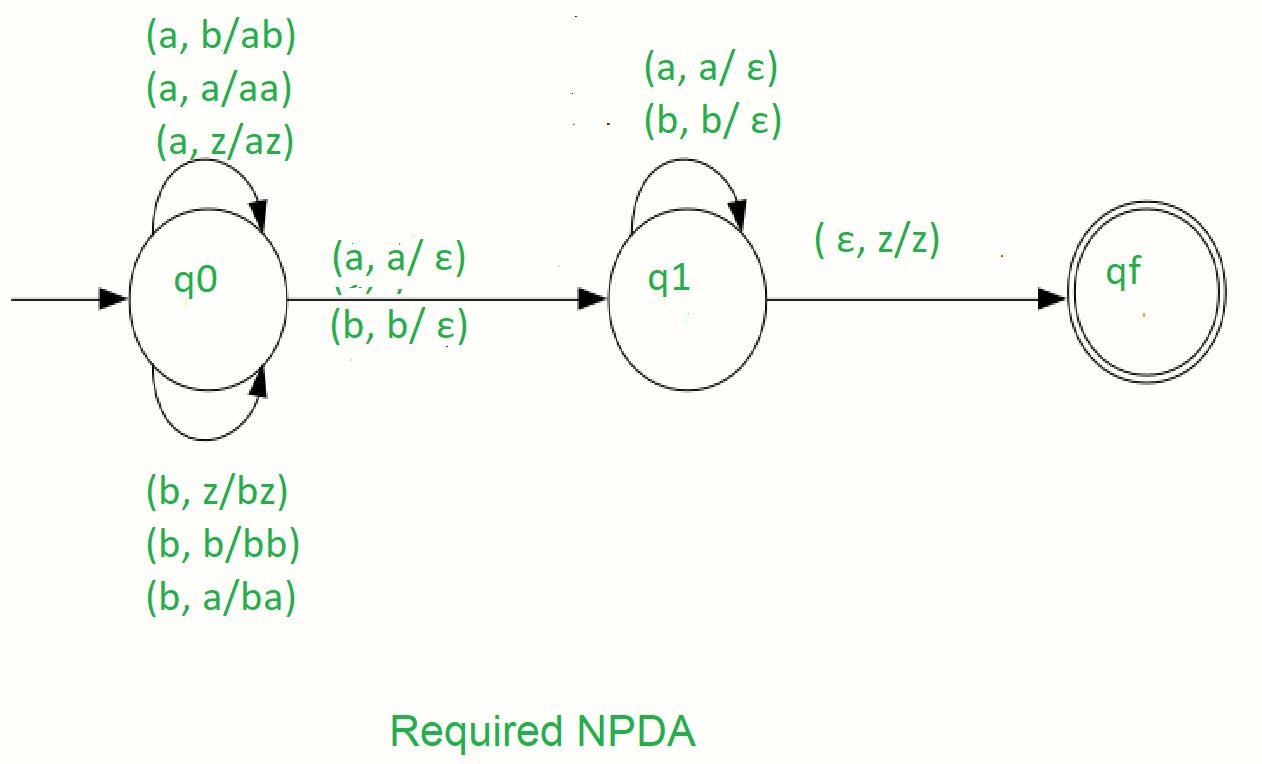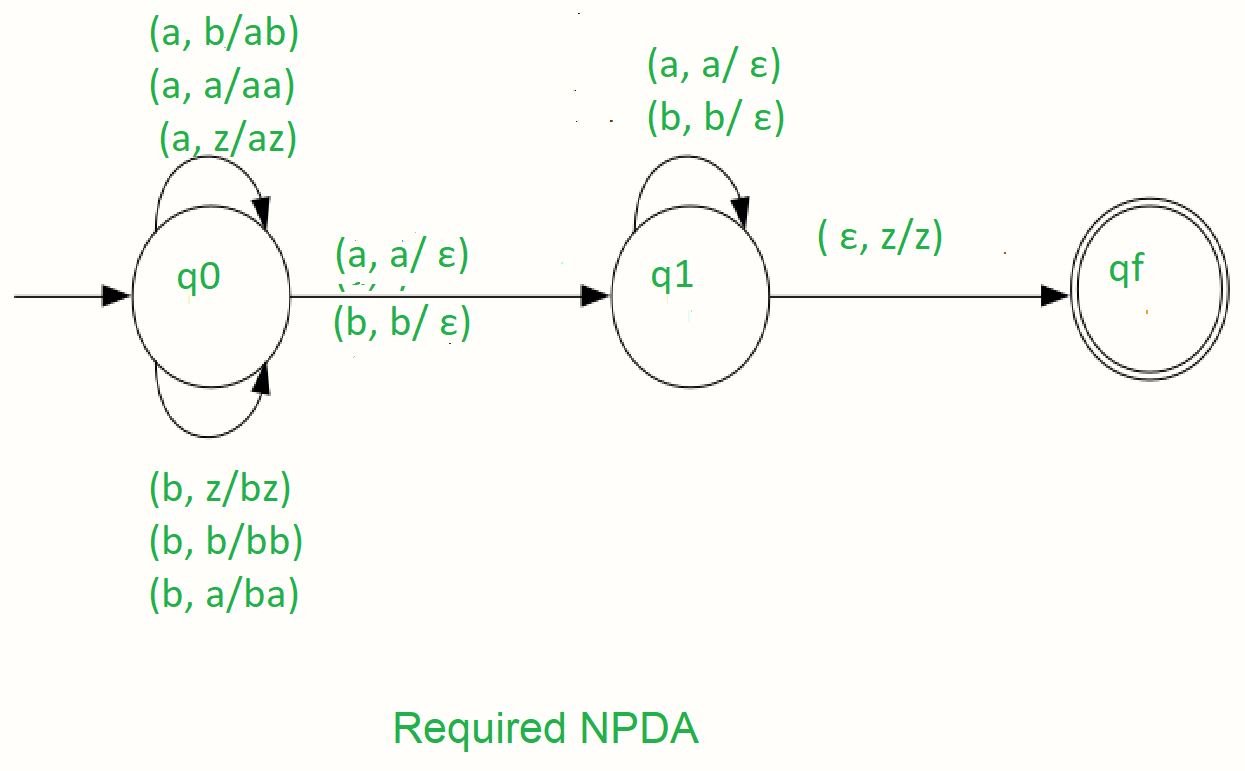# NPDA for accepting the language L = {wwR | w ∈ (a,b)*}

Prerequisite – Pushdown Automata, Pushdown Automata Acceptance by Final State
Problem: Design a non deterministic PDA for accepting the language L = {wwR w ∈ (a, b)*}, i.e.,

L = {aa, bb, abba, aabbaa, abaaba, ......}

Explanation:
In this type of input string, at one input has more than one transition states, hence it is called non deterministic PDA and input string contain any order of ‘a’ and ‘b’. Each input alphabet has more than one possibility to move next state. And finally when stack is empty then the string is accepted by the NPDA. In this NPDA we used some symbol which are given below:

Γ = { a, b, z }

Where, Γ = set of all the stack alphabet
z = stack start symbol
a = input alphabet
b = input alphabat

Approach used in the construction of PDA –
As we want to design a NPDA, thus every times ‘a’ or ‘b’ comes then either push into the stack or move into the next state. It is dependent on a string.When we see the input alphabet which is equal to the top of stack then that time pop operation apply on the stack and move to next step.
So, at the end if the stack becomes empty then we can say that the string is accepted by the PDA.

STACK Transiton Function(q0, a, z)(q0, az)(q0, a, a)(q0, aa)(q0, b, z)(q0, bz)(q0, b, b)(q0, bb)(q0, a, b)(q0, ab)(q0, b, a)(q0, ba)(q0, a, a)(q1, ∈)(q0, b, b)(q1, ∈)(q1, a, a)(q1, ∈)(q1, b, b)(q1, ∈)(q1, ∈, z)(qf, z)


Where, q0 = Initial state
qf = Final state
∈ = indicates pop operationSo, this is our required non deterministic PDA for accepting the language L = {wwR w ∈ (a, b)*}

Example:
We will take one input string: “abbbba”.

• Scan string from left to right
• First input is ‘a’ and follow the rule:
• on input ‘a’ and STACK alphabet Z, push the two ‘a’s into STACK as : (a, Z/aZ) and state will be q0
• on input ‘b’ and STACK alphabet ‘a’, push the ‘b’ into STACK as : (b, a/ba) and state will be q0
• on input ‘b’ and STACK alphabet ‘b’, push the ‘b’ into STACK as : (b, b/bb) and state will be q0
• on input ‘b’ and STACK alphabet ‘b’ (state is q1), pop one ‘b’ from STACK as : (b, b/∈) and state will be q1
• on input ‘b’ and STACK alphabet ‘b’ (state is q1), pop one ‘b’ from STACK as : (b, b/∈) and state will be q1
• on input ‘a’ and STACK alphabet ‘a’ and state q1, pop one ‘a’ from STACK as : (a, a/∈) and state will remain q1
• on input ∈ and STACK alphabet Z, go to final state(qf) as : (∈, Z/Z)

So, at the end the stack becomes empty then we can say that the string is accepted by the PDA.

Problem:
Design a deterministic PDA for accepting the language L = { wcwR w ∈ (a, b)*}, i.e.,

{aca, bcb, abcba, abacaba, aacaa, bbcbb, .......}

In each of string, sub string which are present in left side of c is reverse of sub string which is present right side of c.

Explanation :
Here we need to maintain string in such way that, substring which is present in the left side of c is exactly reverse substring which is the right side of c. For doing this we used a stack.In string ‘a’ and ‘b’ are present any order and ‘c’ is comes only one times. When ‘c’ comes then after pop operation is start into the stack. And when a stack is empty then language is accepted.

Γ = {a, b, z}

Where, Γ = set of all the stack alphabet
z = stack start symbol
a = input alphabate
b = input alphabate

Approach used in the construction of PDA:
As we want to design PDA In every time when ‘a’ or ‘b’ comes we push into the stack and stay on same state q0. And when ‘c’ comes then we move to the next state q1 without pushing ‘c’ into the stack. And after when comes an input which is the same as the top of the stack then pop from the stack and stay on the same state. POP operation is performed until the input string is end. Finally when the input is ∈ then move to the final state qf.
When if the stack will become empty then language is accepted.

Where, q0 = Initial state
qf = Final state
z = stack start symbol
∈= indicates pop operation

Stack transition functions:(q0, a, z)(q0, az)(q0, a, a)(q0, aa)(q0, b, z)(q0, bz)(q0, b, b)(q0, bb)(q0, a, b)(q0, ab)(q0, b, a)(q0, ba)(q0, c, a)(q1, a)(q0, c, b)(q1, b)(q1, a, a)(q1, ∈)(q1, b, b)(q1, ∈)(q1, ∈, z)(qf, z) 

Where, q0 = Initial state
qf = Final state
∈ = indicates pop operationSo, this is our required deterministic PDA for accepting the language,

L = { wcwR w ∈ (a, b)*}My Personal Notes arrow_drop_upCheck out this Author's contributed articles.

If you like GeeksforGeeks and would like to contribute, you can also write an article using contribute.geeksforgeeks.org or mail your article to contribute@geeksforgeeks.org. See your article appearing on the GeeksforGeeks main page and help other Geeks.

Please Improve this article if you find anything incorrect by clicking on the "Improve Article" button below.

Article Tags :

Be the First to upvote.

Please write to us at contribute@geeksforgeeks.org to report any issue with the above content.